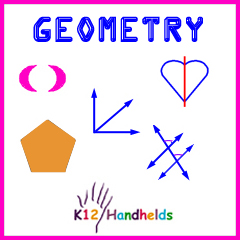Portal, AZ
www.k12handhelds.com

Copyright © 2008 by K12 Handhelds, Inc. License CC-by,Developed in conjunction with Wayne Township School System.

# Illustrated Glossary

## A-E

acute angle – an angle that is less than 90°
An acute angle is smaller than a perfectly square corner.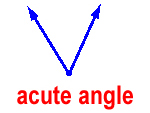acute triangle – a triangle with three acute angles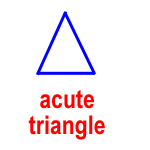adjacent angles – two angles that share a vertex (the point where two sides meet) and a common side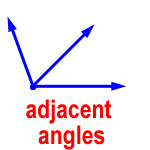angle of rotation – when rotating a shape, the angle and direction of rotation

The direction is given as clockwise or counter-clockwise.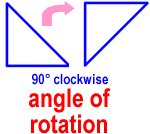center of rotation – the fixed point a figure is rotated around

complementary angles – two angles that have a sum of 90°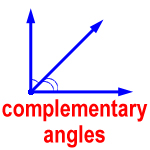congruent angles – two angles that have the same measurement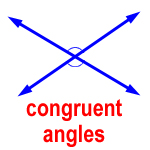congruent polygonspolygons that have the same shape and the same size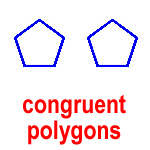congruent sides – sides that are the same length

corresponding angles – two congruent angles on the same side of a line that crosses two parallel lines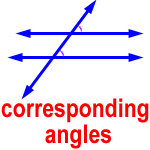equilateral triangle – a triangle with three congruent sides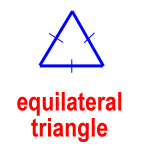## F-Q

heptagon – a polygon with seven sides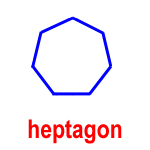hexagon – a polygon with six sides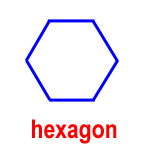image – the new figure or shape formed after a transformation

intersecting lines – two lines that meet at a point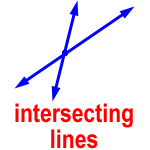isosceles triangle – a triangle with two congruent sides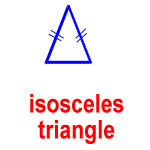line of reflection – the line a shape is reflected or flipped over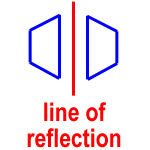line of symmetry – the line that divides something with line symmetry

line symmetry – when a figure or shape can be divided by a line so that the part on each side is a mirror image of the part on the other side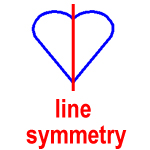obtuse angle – an angle that is more than 90° but less than 180°
An obtuse angle is bigger than a perfectly square corner, but smaller than a straight line.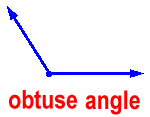obtuse triangle – a triangle with one obtuse angle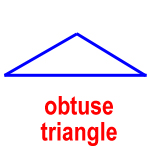octagon – a polygon with eight sides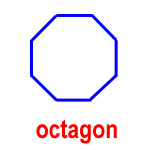parallel lines – two lines in the same plane that are the same distance apart at all points and never meet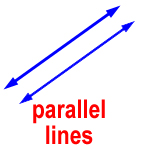parallelogram – a quadrilateral with two pairs of parallel sides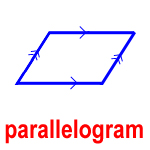pentagon – a polygon with five sides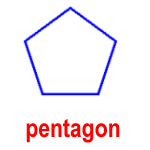perpendicular lines – two lines that meet to form four right angles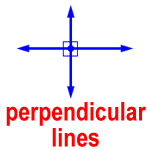plane – a flat surface that extends in all directions without endpolygon – a closed plane figure made of connected line segments
Triangles, squares, and rectangles are examples of polygons. They are all closed shapes.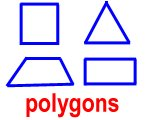quadrilateral – a polygon with four sides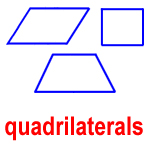## R-V

reflection – a flip; a way of moving a shape across a line, producing a mirror image, like flipping a pancake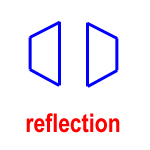regular polygon – a polygon with all congruent sides and all equal angles

rhombus – a parallelogram with four congruent sides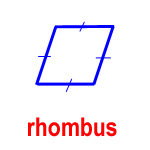right angle – an angle that is exactly 90°
A right angle makes a perfectly square corner.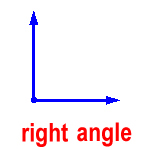right triangle – a triangle with one right angle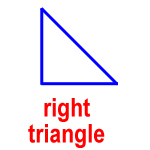rotation – a turn; turning or spinning a shape, like a doing a cartwheel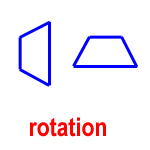rotational symmetry – when a figure is turned 180° or less and produces a figure that is identical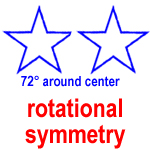scalene triangle – a triangle with no congruent sides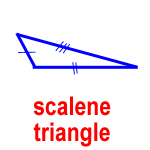similar polygonspolygons that have the same shape but different sizes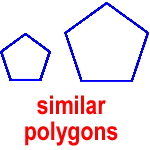straight angle – an angle that is exactly 180°
A straight angle makes a perfectly straight line.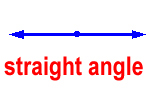supplementary angles – two angles that have a sum of 180°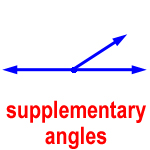transformation – a movement of a shape on a plane; a change in a shape; a way of mapping the shape onto another space
Examples of transformations are translations, reflections, and rotations.

translation – a slide; moving a shape along a line or scooting it over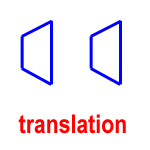trapezoid – a quadrilateral with one pair of parallel sides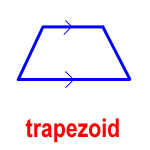vertical angles – the opposite angles formed when two lines cross
Vertical angles are always congruent angles. They have the same measurement.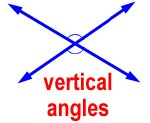# Practice

1. Match the type of angle with its picture.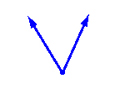acute obtuse right straight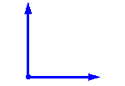acute obtuse right straight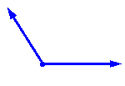acute obtuse right straight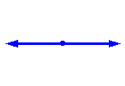acute obtuse right straight

Check the box or boxes that describe each set of angles. There may be more than one correct answer.

 2.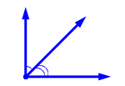supplementary complementary adjacent vertical congruent

 3.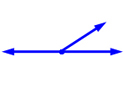congruent complementary adjacent supplementary vertical

 4.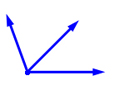congruent complementary vertical supplementary adjacent

 5.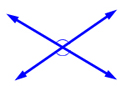vertical adjacent congruent complementary supplementary

6. What are two lines that never meet called?

7. What are two lines that meet to form four right angles called?

8. What is a flat surface that extends with no end?

9. Which two angles are corresponding?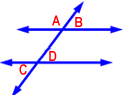A) B and D
B) A and B
C) B and C
D) C and D

10. Which two angles are vertical?A) A and B
B) B and D
C) C and D
D) B and C

11. Which two angles are supplementary?A) C and D
B) A and B
C) B and D
D) B and C

12. Which kind of triangle is this?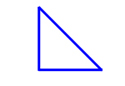13. Which kind of triangle is this?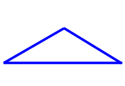14. Which kind of triangle is this?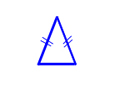15. Which kind of triangle is this?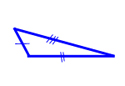16. Which kind of triangle is this?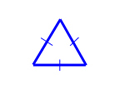17. Which kind of triangle is this?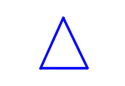Match the polygon with its name.

18.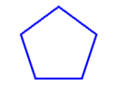19.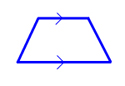20.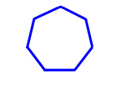21.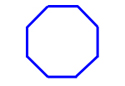22.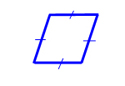23.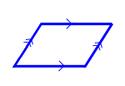24.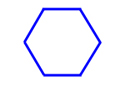25. These two polygons are ___.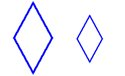A) regular
B) similar
C) congruent

26. These two polygons are ___.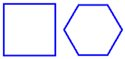A) regular
B) similar
C) congruent

27. These two polygons are ___.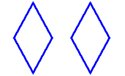A) regular
B) similar
C) congruent

28. The transformation shown here is a ___.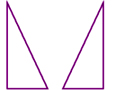29. The transformation shown here is a ___.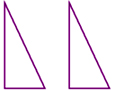30. The transformation shown here is a ___.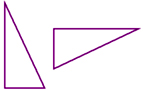31. What is the angle of rotation in this rotation?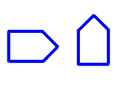32. What is the angle of rotation in this rotation?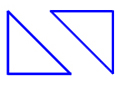33. What is the angle of rotation in this rotation?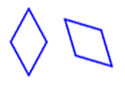34. Does this figure have line symmetry?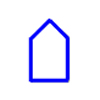A) yes
B) no

35. Does this figure have line symmetry?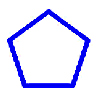A) yes
B) no

36. Does this figure have line symmetry?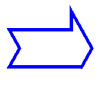A) yes
B) no

37. Does this figure have line symmetry?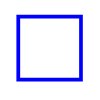A) yes
B) no

38. Does this figure have rotational symmetry?A) yes
B) no

39. Does this figure have rotational symmetry?A) yes
B) no

40. Does this figure have rotational symmetry?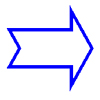A) yes
B) no

41. Does this figure have rotational symmetry?A) yes
B) no